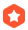# 关灯游戏(ACM/ICPC题)lkrich7 2005-01-19 10:52:26加精

0 1 1 0 1 0
1 0 0 1 1 1
0 0 1 0 0 1
1 0 0 1 0 1
0 1 1 1 0 0

0 1 0 0 1 0
1 1 1 0 1 1
0 0 0 0 0 1
1 0 0 1 0 1
0 1 1 1 0 0

1.矩阵的状态与按开关的顺序无关
2.如果某个开关按下了两次，那么就相当于取消了第一次的操作，也就是说没有开关需要按超过1次

http://acm.pku.edu.cn/JudgeOnline/showproblem?problem_id=1222

...全文
1236 9 打赏 收藏 举报

9 条回复

mmmcd 2005-01-26

void process(){
...
for(x=0;x<5;x++)
for(y=0;y<6;y++)
cin>>temp; -> cin>>temp[x][y];
...
}

void output(){
...
cout<<an[x]; -> cout<<ans[x];
...
}
• 打赏
• 举报

mmmcd 2005-01-26
#include<iostream.h>
#include<memory.h>
void process();//求解的过程
void press(int,int);//处理按键的过程
void output();//输出结果
int lights;//记录灯状态，0灭，1亮
int ans;//记录结果，若在x行y列点击，ans[x-1][y-1]=1
int main(){ //主函数
int n; cin>>n;
for(int i=1;i<=n;i++){
cout<< "PUZZLE #" <<i<<endl;
process(); //整个求解过程
}
}

void press(int x,int y)
{//处理按键的过程
ans[x][y]=1;//记录操作
lights[x][y]=1-lights[x][y];//0->1，1->0
if(x>0)lights[x-1][y]=1-lights[x-1][y];
if(y>0)lights[x][y-1]=1-lights[x][y-1];
if(x<4)lights[x+1][y]=1-lights[x+1][y];
if(y<5)lights[x][y+1]=1-lights[x][y+1];
}

void process(){
int x,y,z,temp;
for(x=0;x<5;x++)
for(y=0;y<6;y++)cin>>temp;
for(z=0;z<64;z++){//外循环,枚举64个状态
memcpy(lights,temp,sizeof(lights));
memset(ans,0,sizeof(ans));//初始化
for(y=0;y<6;y++)
if(z & (1<<y))/*如果z右起第y个bit位是‘1’则在第1行y列点击*/
press(0,y);//枚举第一行的64种操作。
for(x=1;x<5;x++)
for(y=0;y<6;y++)
if(lights[x-1][y]==1)press(x,y);/*就是刚才所说的规则*/
for(y=0;y<6;y++)if(lights[y]==1)break; //判断最后一行是否全灭
if(y>=6){ output();break; } //是,输出结果，结束搜索
}
}
void output(){
int x,y;
for(x=0;x<5;x++){
cout<<an[x];
for(y=1;y<6;y++)cout<<' '<<ans[x][y];
cout<<endl;
}
}
• 打赏
• 举报

LeeMaRS 2005-01-19
ZJU 1354 - Extended Lights Out - 00:00.00 52K - 枚举 + 判断
http://acm.zju.edu.cn/show_problem.php?pid=1354
AC 1次

• 打赏
• 举报

NowCan 2005-01-19

• 打赏
• 举报

• 打赏
• 举报

mathe 2005-01-19

C(k)=(0,0,...,1,0,....,1,...,0)

X+C(k) (mod 2)

X+C(k1)+C(k2)+....+C(ks) (mod 2)=O=(0,0,0,...,0)

X+Y*C (mod 2)=O

Y*C (mod 2)=X

• 打赏
• 举报

mathe 2005-01-19
m*n的矩阵，在二阶域上解一个(m*n)阶的线性方程组就可以了
• 打赏
• 举报2017ACM-ICPC 西安赛区总结
~~~~~~~周四晚上出发去西安，因为学校只能报硬座，再加上学弟们那段时间生活费比较紧张以及我们坐的那趟火比较快的缘故，总之，那天晚上坐的是硬座，第一次坐硬座，没想到这么难受：第一点是硬座晚上不关灯，你第七届ACM程序设计竞赛 (SDIBT)dp 46（再做一遍）
Robberies http://acm.hdu.edu.cn/showproblem.php?pid=2955 背包;第一次做的时候把概率当做背包(放大100000倍化为整数):在此范围内最多能抢多少钱 最脑残的是把总的概率以为是抢N家银行的概率之和… 把状态...80目+AC代码汇总 ~ 南阳 NYOJ总结：WHUACM 2007暑假招新笔试（附原，有许多经典的面试目）